In :
```import pandas as pd
import numpy as np
```
In [ ]:
```# Take few samples for the visualization
sample_fbcheckin_train_tbl = fbcheckin_train_tbl[:10000].copy()
```
In :
```df = pd.read_csv('train.csv', index_col='row_id')
```
In :
```df.head()
```
Out:
x y accuracy time place_id
row_id
0 0.7941 9.0809 54 470702 8523065625
1 5.9567 4.7968 13 186555 1757726713
2 8.3078 7.0407 74 322648 1137537235
3 7.3665 2.5165 65 704587 6567393236
4 4.0961 1.1307 31 472130 7440663949
In :
```print('Reading train data')
print('\nSize of training data: ' + str(df.shape))
print('Columns:' + str(df.columns.values))
print('Number of places: ' + str(len(list(set(df['place_id'].values.tolist())))))
print('\n')
print('dtypes')
print('\n')
print(df.dtypes)
print('\n')
print('Info: ')
print('\n')
print(df.info)
print('Shape: ')
print('\n')
print(df.shape)
print('\n')
print('numerical columns statistcs')
print('\n')
print(df.describe())
```
```Reading train data

Size of training data: (29118021, 5)
Columns:['x' 'y' 'accuracy' 'time' 'place_id']
Number of places: 108390

dtypes

x           float64
y           float64
accuracy      int64
time          int64
place_id      int64
dtype: object

Info:

<bound method DataFrame.info of                x       y  accuracy    time    place_id
row_id
0         0.7941  9.0809        54  470702  8523065625
1         5.9567  4.7968        13  186555  1757726713
2         8.3078  7.0407        74  322648  1137537235
3         7.3665  2.5165        65  704587  6567393236
4         4.0961  1.1307        31  472130  7440663949
5         3.8099  1.9586        75  178065  6289802927
6         6.3336  4.3720        13  666829  9931249544
7         5.7409  6.7697        85  369002  5662813655
8         4.3114  6.9410         3  166384  8471780938
9         6.3414  0.0758        65  400060  1253803156
10        2.0173  4.8627         6   21353  8684462954
11        8.7101  2.9442        73  153493  2159916487
12        0.8829  1.3445        64  574488  7652380351
13        2.4336  8.0600        62  238054  8234363596
14        6.1550  1.9774         8  325411  2272949794
15        7.6219  9.6208        65  321519  4740742194
16        3.2494  3.2096        75  777982  2123587484
17        0.7084  8.9051        69  320633  8016758016
18        2.7256  1.0135        54    6249  8936085695
19        4.2683  1.8238        70  411437  2778700985
20        5.3298  6.3457        52  736113  4845908305
21        4.2830  3.1855        62  500479  3938338894
22        9.6349  1.2462       172  181701  9784464752
23        7.4492  8.9950        17  726403  1874355796
24        1.2837  7.5588         4  245292  9885174082
25        7.6423  6.7629        70  323935  4294512385
26        4.6375  3.4561        67  297623  7766380992
27        4.3794  7.2107       165  727097  2110807282
28        7.2382  4.3998        75   11929  9713229580
29        4.5083  1.8794         2  310734  6163271747
...          ...     ...       ...     ...         ...
29117991  1.4695  4.7915       170   13722  9518416844
29117992  4.3447  3.6639        60  392466  4579210194
29117993  8.7312  4.3808        57  185192  7082695242
29117994  8.1875  5.9198        55  187524  1550124580
29117995  1.3297  2.4151       157  121341  1226687693
29117996  9.6885  6.0283        62  330458  3530972003
29117997  7.6774  9.2865         3    7341  2856274327
29117998  9.1955  5.7141         4  363864  7842684810
29117999  3.1623  6.1656        65  518841  7594986161
29118000  9.8670  4.7670        64  405522  4745521730
29118001  1.6180  7.7528        15  181869  1024290530
29118002  3.5529  9.7930        24  684885  9033567575
29118003  5.5334  4.9625       157  406449  1329555199
29118004  7.0986  7.4478       178  531555  5731650052
29118005  7.0048  7.9702        96  670327  5039310244
29118006  8.8605  2.8834        72  642558  3822809844
29118007  8.1419  6.9827       522  586524  9294668810
29118008  8.7879  9.7045       159  776578  9791467472
29118009  3.4611  3.2491        68  535917  8647480716
29118010  1.4461  5.0805        73  134152  2596462819
29118011  4.4198  9.1936         9  107847  6554476200
29118012  5.1348  1.9838        16  528290  1817075880
29118013  7.1625  1.9283        70  513238  1406544605
29118014  7.4980  3.8432       192   42442  5515553973
29118015  1.6417  9.0446       643  549617  5760372884
29118016  6.5133  1.1435        67  399740  8671361106
29118017  5.9186  4.4134        67  125480  9077887898
29118018  2.9993  6.3680        67  737758  2838334300
29118019  4.0637  8.0061        70  764975  1007355847
29118020  7.4523  2.0871        17  102842  7028698129

[29118021 rows x 5 columns]>
Shape:

(29118021, 5)

numerical columns statistcs

x             y      accuracy          time      place_id
count  2.911802e+07  2.911802e+07  2.911802e+07  2.911802e+07  2.911802e+07
mean   4.999770e+00  5.001814e+00  8.284912e+01  4.170104e+05  5.493787e+09
std    2.857601e+00  2.887505e+00  1.147518e+02  2.311761e+05  2.611088e+09
min    0.000000e+00  0.000000e+00  1.000000e+00  1.000000e+00  1.000016e+09
25%    2.534700e+00  2.496700e+00  2.700000e+01  2.030570e+05  3.222911e+09
50%    5.009100e+00  4.988300e+00  6.200000e+01  4.339220e+05  5.518573e+09
75%    7.461400e+00  7.510300e+00  7.500000e+01  6.204910e+05  7.764307e+09
max    1.000000e+01  1.000000e+01  1.033000e+03  7.862390e+05  9.999932e+09
```

OK: so we have ~29 million records.

A few notes:

1. row_id seems to be … a row ID. It is TRUE that the number of unique row_ids is the same as the number of rows in the data frame.
2. x is presumably bounded between [0, 10] as the x-axis on the 10-km square.
3. y looks to be the same as x, just the other dimension.
4. accuracy is intersting: it’s all over the place. The smallest value is 1.00; the biggest value is 1,033.00. We’ll have to look into that.
5. time has no units. Since Facebook notes that time an accuracy are “intentionally left vague in their definitions.”, we will have to look into that.
6. place_id is probably a unique identifier. There 108390 unique values.

Let’s start by examining the “vague” variables.

Accuracy We already know that accuracy isn’t exactly defined. From first principles, we could think of it a few ways –

Error on some arbitary scale. This seems unlikely, since the max is > 1,000. Error on the same scale as x and y. Now, this could be an estimated radius (with the x and y values as the center); either normal or squared. Since we have a lot of data, and we’re running this in Kaggle scripts, we can randomly sample 1% of the data and look at the data. The pattern will (almost certainly) be the same.

In :
```import matplotlib.pyplot as plt
%matplotlib inline
```
In :
```# the histogram of the data
n, bins, patches = plt.hist(df['accuracy'], 50, normed=1, facecolor='r', alpha=0.75)
```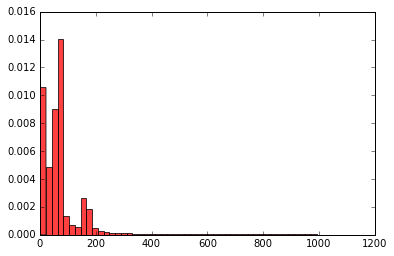It looks like we have three peaks. Do we think there are underlying parameters in the simulation at these peaks?

We might also think that there are different measures of accuracy at different locations.

Let’s see how even the accuracy is over x and y.

In :
```# change outlier point symbols horizontal boxes
plt.figure()
plt.boxplot(df['accuracy'],0, 'o',0)
plt.show()
```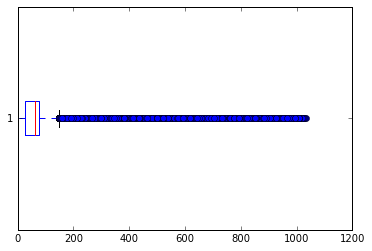In [ ]:
```from scipy.stats import kurtosis,skew
kurtosis(df['accuracy'])
skew(df, axis=0, bias=True)
```
Out[ ]:
```array([ -8.96831633e-16,  -1.20295052e-03,  -3.76966172e-04,
4.59157372e+00,  -1.35186650e-01,  -3.47188384e-03])```
In :
```df[df.x<0.1].x.hist(bins=100)

plt.xlabel('x')
plt.ylabel('Number of checkins');
```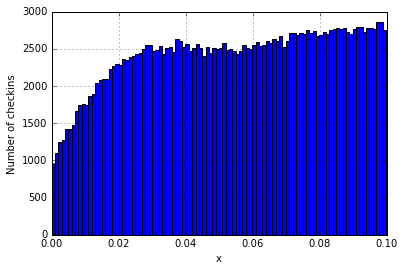In [ ]:
```#3D plot grouping the data into rounded off x and y
from mpl_toolkits.mplot3d import Axes3D
plt.rcParams['figure.figsize'] = (16,5)
```
In [ ]:
```df['grid_x'] = df.x.apply(lambda x: round(x))
df['grid_y'] = df.y.apply(lambda y: round(y))
grouped_grid = df.groupby(['grid_x', 'grid_y'])
grid_freq = grouped_grid.size().reset_index()
```
In [ ]:
```fig = plt.figure()

xpos = grid_freq.grid_x
ypos = grid_freq.grid_y
zpos = np.zeros(len(grouped_grid))

dx = np.ones(len(grouped_grid))
dy = np.ones(len(grouped_grid))
dz = grid_freq

ax1.bar3d(xpos, ypos, zpos, dx, dy, dz, color='#00ceaa')

plt.show()
```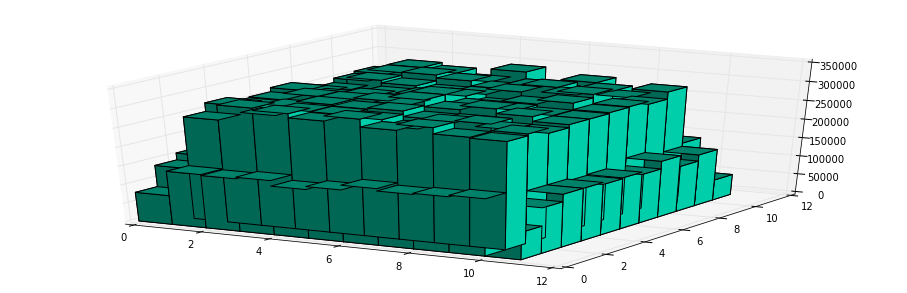I didn’t see many people experimenting with the accuracy so I was curious if I could find anything from it. My goal was to find some sort of relationship between accuracy and the variation in x and y. The purpose of this was so that I could eventually make a way to artificially populate the data set with ‘ghosts’ of the same data point in a localized radius (obtained from this relationship between accuracy and distance).

In :
```mean_train_data = df.groupby('place_id').mean()
std_train_data = df.groupby('place_id').std()

acc_df = pd.concat([mean_train_data['accuracy'],std_train_data['x'],std_train_data['y']], axis=1)
acc_df.rename(columns={'accuracy':'mean_accuracy','x':'std_x','y':'std_y'}, inplace=True)

acc_df.fillna(0, inplace=True)
```
In :
```p = plt.hist(acc_df.mean_accuracy, bins=np.arange(min(acc_df.mean_accuracy), max(acc_df.mean_accuracy) + 1, 1))
plt.xlabel('Mean Accuracy')
plt.ylabel('Count')
plt.title('Counts of mean accuracy for places')

plt.show()
```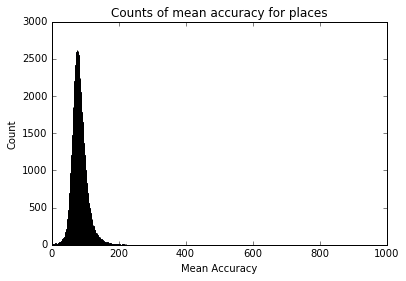In :
```p = plt.hist(acc_df.std_x, bins=np.arange(min(acc_df.std_x), max(acc_df.std_x) + .01, .01))
plt.xlabel('Std(y)')
plt.ylabel('Count')
plt.title('Counts of std(x) for places')

plt.show()
```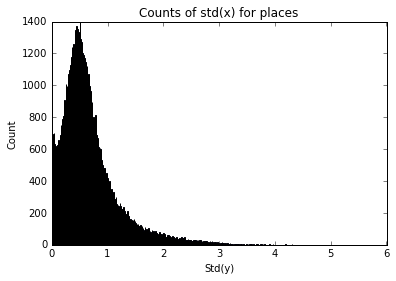In :
```p = plt.hist(acc_df.std_y, bins=np.arange(min(acc_df.std_y), max(acc_df.std_y) + .001, .001))
plt.xlabel('Std(y)')
plt.ylabel('Count')
plt.title('Counts of std(y) for places')

plt.show()
```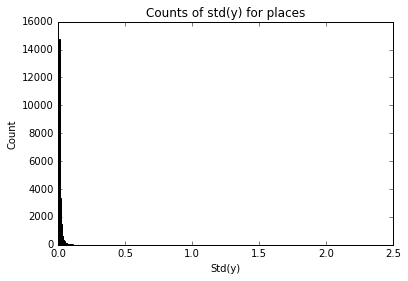In :
```p = plt.scatter(acc_df.mean_accuracy,acc_df.std_x)
plt.xlabel('Mean Accuracy')
plt.ylabel('Std(x)')
plt.title('std(x) vs Mean accuracy for each place')

plt.show()
```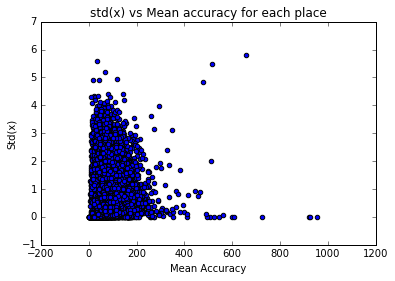Some outliers in this graph but for the most part this is about what was expected, higher accuracy generally leads to lower deviation in distance and the opposite for lower accuracy. The variation in this data is larger than what would be ideal, which could cause some distance approximations to be very inaccurate but it is a start.

In :
```p = plt.scatter(acc_df.mean_accuracy, acc_df.std_y)
plt.xlabel('Mean Accuracy')
plt.ylabel('Std(y)')
plt.title('std(y) vs mean accuracy for each place')

plt.show()
```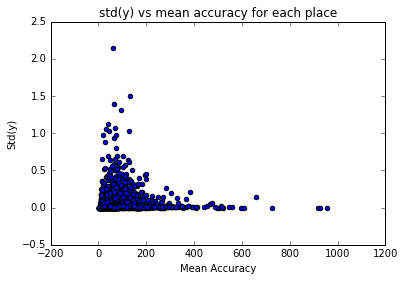In :
```df.time.describe()
```
Out:
```count    2.911802e+07
mean     4.170104e+05
std      2.311761e+05
min      1.000000e+00
25%      2.030570e+05
50%      4.339220e+05
75%      6.204910e+05
max      7.862390e+05
Name: time, dtype: float64```
In :
```range = df.time.max() - df.time.min()
print(range)
```
```786238
```
In :
```print('Calculate hour, weekday, month and year for train and test')
df['hour'] = (df['time']//60)%24+1 # 1 to 24
df['weekday'] = (df['time']//1440)%7+1
df['month'] = (df['time']//43200)%12+1 # rough estimate, month = 30 days
df['year'] = (df['time']//525600)+1
```
```Calculate hour, weekday, month and year for train and test
```
In :
```print('group by place_id and get count')
places = df[['place_id', 'time']].groupby('place_id').count()
```
```group by place_id and get count
```
In :
```import seaborn as sns

places.rename(columns={'time': 'count'}, inplace=True)

print('plot weekday Vs hour for 6 place_ids with highest counts')
plt.figure(1, figsize=(14,10))
placeindex = places['count'].sort_values(ascending=False)[:6]
for (i, placeid) in enumerate(placeindex.index):
ax = plt.subplot(2,3,i+1)
df_place = df.query('place_id == @placeid')
# df_place = train.query('place_id == @placeid and year==1') # to separate by year
sns.kdeplot(df_place.weekday, df_place.hour, shade=True, ax = ax)
plt.title("place_id " + str(placeid))
ax.set(xlim=(0, 8))
ax.set(ylim=(0, 25))
```
```plot weekday Vs hour for 6 place_ids with highest counts
```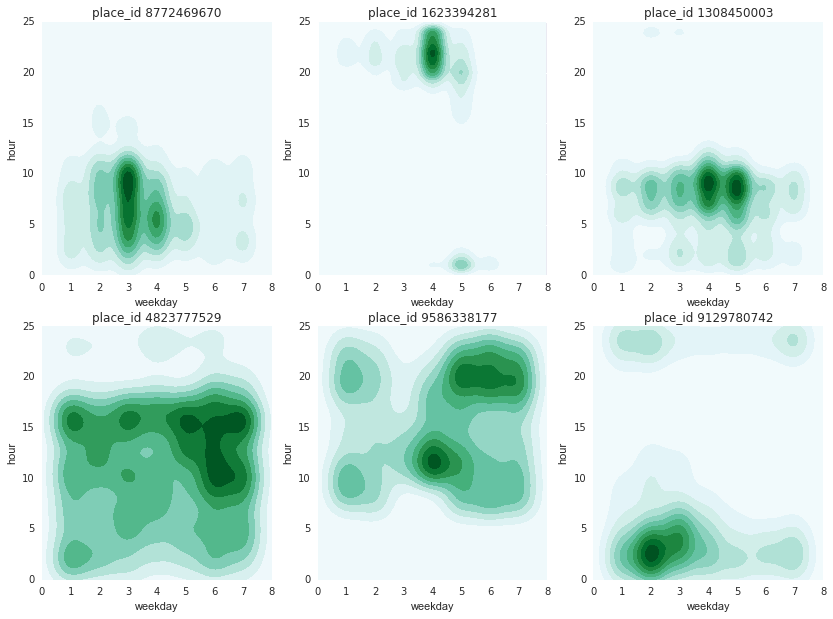Kdes for weekday Vs hour for 6 place ids with the highest counts. The plots show a preference for certain hours and weekdays for each place id. Maybe weekends and weekdays and holidays can be separated if more place ids are analyzed.

In :
```print('plot weekday Vs month for 6 place_ids with highest counts')
plt.figure(2, figsize=(14,10))
placeindex = places['count'].sort_values(ascending=False)[:6]
for (i, placeid) in enumerate(placeindex.index):
df_place = df.query('place_id == @placeid and year==1')
ax = plt.subplot(2,3,i+1)
plt.title("place_id " + str(placeid))
```
```plot weekday Vs month for 6 place_ids with highest counts
```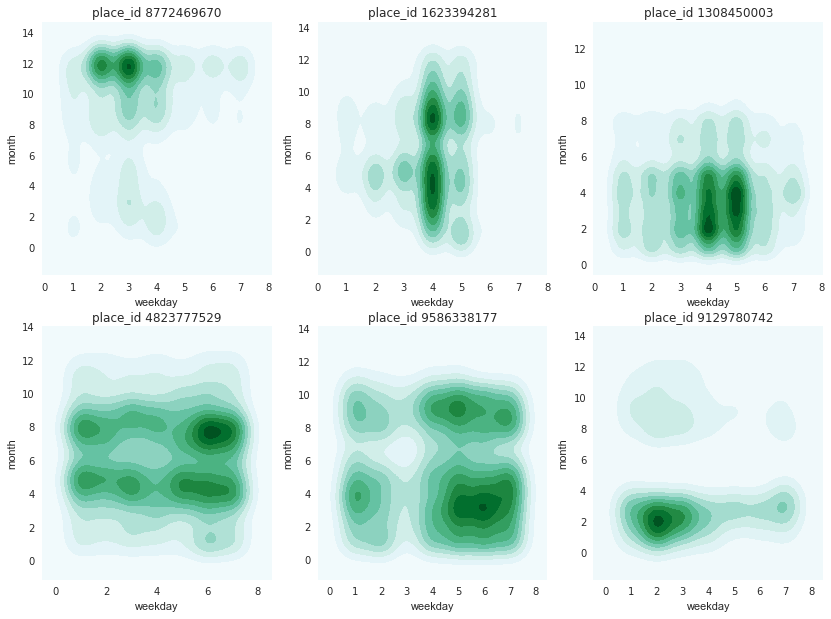Estimate the autocorrelation of all of the timestamps to see if there are any repeating patterns

In :
```# Pull out timestamps
#Remove single-dimensional entries from the shape of an array.
times = np.squeeze(df.as_matrix(columns=['time']))
```
In :
```sns.distplot(df.time.sample(frac=0.01))
```
Out:
`<matplotlib.axes._subplots.AxesSubplot at 0x1b9cd589198>`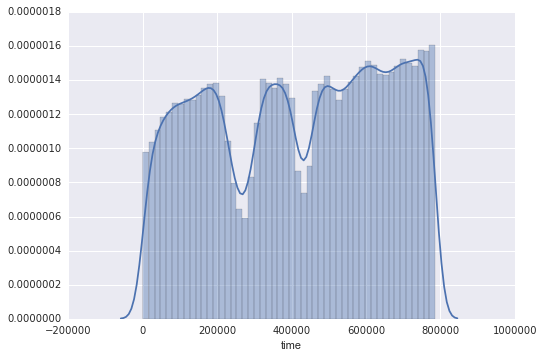Simply looking at the KDE distribution of time does not provide much information. The only thing to notice are two valleys that probably indicate some kind of seasonality.

FFT (Fast Fourier Transform) refers to a way the discrete Fourier Transform (DFT) can be calculated efficiently, by using symmetries in the calculated terms. The symmetry is highest when n is a power of 2, and the transform is therefore most efficient for these sizes.

In :
```ts_count = df.time.value_counts().sort_index()
ts_fft = np.fft.fft(ts_count.tolist())
print(ts_fft)
```
```[ 29118021.00000000      +0.j           1332210.21294901+1834917.83093776j
-309288.15979606  +95686.06929086j ...,
-1047447.59552521  -55852.49332303j   -309288.15979606  -95686.06929086j
1332210.21294902-1834917.83093777j]
```
In :
```import matplotlib as mpl
def discrete_cmap(N, base_cmap=None):
"""Create an N-bin discrete colormap from the specified input map"""
# By Jake VanderPlas

# Note that if base_cmap is a string or None, you can simply do
#    return plt.cm.get_cmap(base_cmap, N)
# The following works for string, None, or a colormap instance:

base = plt.cm.get_cmap(base_cmap)
color_list = base(np.linspace(0, 1, N))
cmap_name = base.name + str(N)
return base.from_list(cmap_name, color_list, N)

def getSamplePlaces(numberOfPlaces=1):

return train[train.place_id.isin((train.place_id).unique()[1:numberOfPlaces+1])]
def getSamplePlace(numberOfPlaces=1):

return train[train.place_id==(train.place_id).unique()[numberOfPlaces]]

def normalize(series):
return (series-min(series))/(max(series)-min(series))

train=df
sample=getSamplePlaces(30)
norm = mpl.colors.Normalize(vmin=min(sample.place_id),vmax=max(sample.place_id))
cp=plt.cm.get_cmap('jet')
cp=discrete_cmap(30,cp)
cols=cp(norm(sample['place_id']))
cols[:,3]=normalize(sample['accuracy'])
fig = plt.figure()
plt.xlabel('x')
plt.ylabel('y')
ax.set_zlabel('time')
plt.show()

#plt.savefig('output.png')
```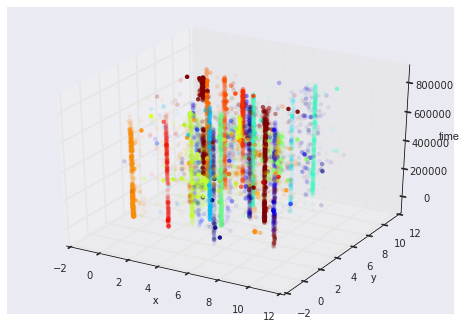In :
```df_test = pd.read_csv("test.csv")
```
In :
```# Sample them for quicker visualisations
df_train_sample = df.sample(n=1000000)
df_test_sample = df_test.sample(n=1000000)
```

### Part 2 – Quick visualisations

Let’s start with some basic histograms, showing the distribution of accuracy and time.

In :
```counts1, bins1 = np.histogram(df["accuracy"], bins=50)
binsc1 = bins1[:-1] + np.diff(bins1)/2.

counts2, bins2 = np.histogram(df_test["accuracy"], bins=50)
binsc2 = bins2[:-1] + np.diff(bins2)/2.

plt.figure(0, figsize=(14,4))

plt.subplot(121)
plt.bar(binsc1, counts1/(counts1.sum()*1.0), width=np.diff(bins1))
plt.grid(True)
plt.xlabel("Accuracy")
plt.ylabel("Fraction")
plt.title("Train")

plt.subplot(122)
plt.bar(binsc2, counts2/(counts2.sum()*1.0), width=np.diff(bins2))
plt.grid(True)
plt.xlabel("Accuracy")
plt.ylabel("Fraction")
plt.title("Test")

plt.show()
```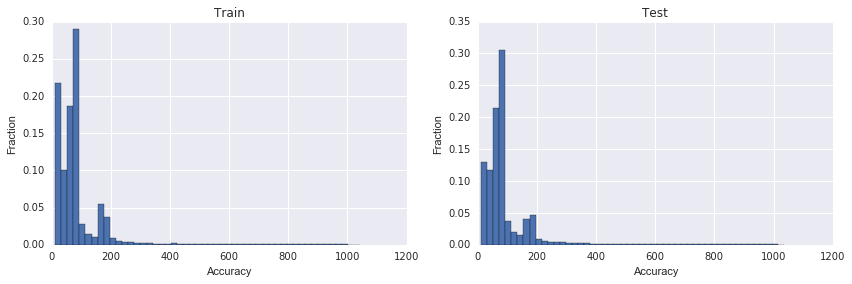The two dips of time in training set are curious, if looking at counts per unit time they might need to be normalised.

Another thing we can look at is how frequently different locations appear.

This site uses Akismet to reduce spam. Learn how your comment data is processed.